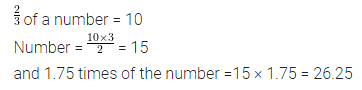# ML Aggarwal Class 7 Solutions for ICSE Maths Chapter 2 Fractions and Decimals Ex 2.6

## ML Aggarwal Class 7 Solutions for ICSE Maths Chapter 2 Fractions and Decimals Ex 2.6

Question 1.
Find the following:
(i) 2.7 × 4
(ii) 2.71 × 5
(iii) 2.5 × 0.3
(iv) 2.3 × 4.35
(v) 238.06 × 7.5
(vi) 0.79 × 32.4
(vii) 1.07 × 0.02
(viii) 10.05 × 1.05
Solution: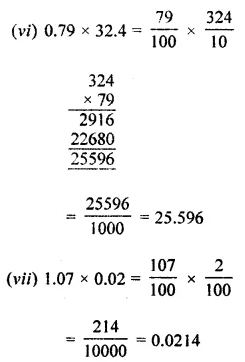Question 2.
find the following:
(i) 10.8 ÷ 4
(ii) 126.35 ÷ 7
(iii) 22.5 ÷ 1.5
(iv) 4.28 ÷ 0.02
(v) 3.645 ÷ 1.35
(vi) 0.728 ÷ 0.04
(vii) 13.06 ÷ 0.08
(viii) 58.635 ÷ 4.5
Solution: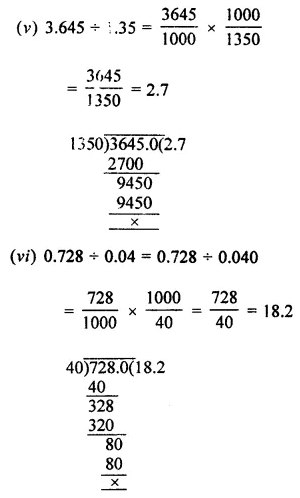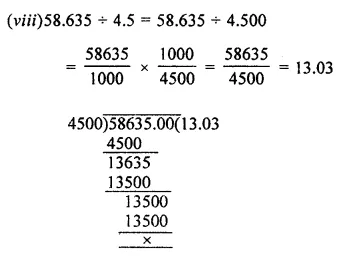Question 3.
Multiply each of the following numbers by 10, 100 and 1000 (orally):
(i) 5.9
(ii) 3.76
(iii) 0.549
Solution:Question 4.
Divide each of the following numbers by 10, 100 and 1000 (orally):
(i) 4.8
(ii) 38.53
(iii) 128.9
Solution:Question 5.
Find the area of a rectangle whose length is 5.7 cm and breadth is 3.5 cm.
Solution: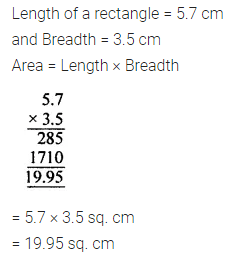Question 6.
The cost of one-metre cloth is ₹ 38.50. Find the cost of 3.6 m cloth.
Solution: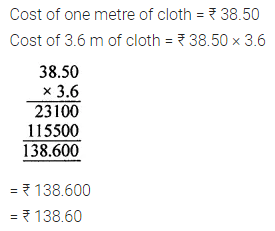Question 7.
A two-wheeler covers a distance of 45.3 km in one litre of petrol. How much distance will it cover in 5.9 litres of petrol?
Solution:Question 8.
If 1 kg of pure milk contains 0.245 kg of fat. How much fat is there in 12.8 kg of milk?
Solution:Question 9.
If ₹ 242.46 are to be distributed among 6 children equally, find the share of each.
Solution: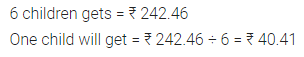Question 10.
A vehicle covers a distance of 43.2 km in 2.4 litres of petrol. How much distance will it cover in one litre of petrol?
Solution: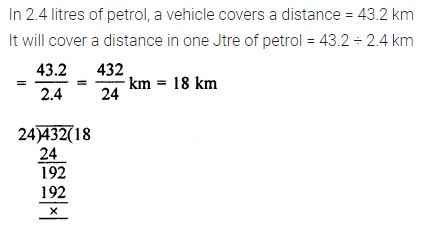Question 11.
How many ice cream cones can be filled from 8.4 litres of ice cream if one cone can be filled with 35 millilitres of ice cream?
Solution: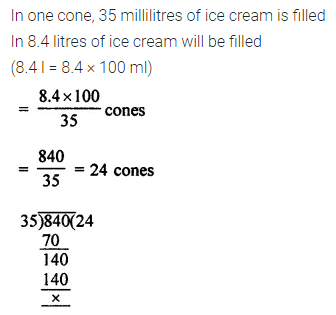Question 12.
If the product of two decimal numbers is 38.745 and one of the numbers is 2.7, find the other.
Solution:Question 13.
If $$\frac { 2 }{ 3 }$$ of a number is 10, then what is 1.75 times of that number?
Solution: